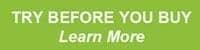# Profit Maximisation Models – Part OneIn 99% of occurrences these traders used some form of profit maximisation strategy and, combined with a bit of luck, just happen to come out on top and we get to read about. Of course for every one of these people there are several thousand that blow up and fall back into obscurity (survivorship bias).

Usually these would-be participants head into contests with very small accounts with the explicit goal of either wiping the account out or becoming the winner. If they wipe out we never hear about them. If they happen to be the lucky one on the day they can build a successful career around it.

Today I’ll cover the first of three profit maximisation strategies. They are designed to grow the account equity as fast as possible but one, specifically Optimal-f, will lead to the complete obliteration of equity.

# Fixed Ratio

This model is often referred to as a position sizing model although it’s really a profit maximisation model. It was developed by Ryan Jones and discussed in his excellent book, ‘The Trading Game’.

The Fixed Ratio approach is mainly used by smaller capitalized traders or those tending to trade a single market, such as a futures market. The key parameter is the Delta which is the dollar amount of profit per contract to increase the number of contracts by 1.

When starting out, the number of contracts to trade will always be 1. As an account increases by the Delta amount, then a second contract is added, and so on. The Delta could be a calculated as a dollar amount or it could be a percentage of account value, but either way Delta remains constant.

Here’s a basic example:

You have a \$10,000 account and trade a single Emini S&P futures contract. You decide that your Delta is \$2,000 which means that for every \$2,000 in new profit you will increase the trade quantity by an extra contract. So under \$12,000 you trade 1 contract, but as soon as the account hits \$12,000 you up the ante to 2 contracts. At \$14,000 you increase the position again to 3 contracts and again to 4 contracts at \$16,000. Should the account slip back you would decrease the number of contracts every \$2,000 by 1 contract.

Here’s the formula:

N = (1/2) [1 + (1+8P / delta) 0.5]

Where,

P = expected profit per trade
delta = the per-contract profit required to increase you holdings by one contract
N = the number of contracts devoted to each trade

One drawback is the actual trade risk is not a factor in the equation.

All that matters is the accumulated profit and the Delta, which is why it’s used for profit maximisation. The Delta determines how quickly the number of contracts are added or subtracted. If you start with 1 contract and set the Delta at 20% gain in account size, then you’re taking on a 100% increase in exposure for a 20% increase in account value.

Fixed Ratio will grow an account fast but the lack of granularity, especially at the front end, will make the ride quite volatile and increase the risk of ruin.

In my next article I’ll cover another technique.